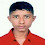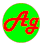# Fourth Semester Syllabus - HYDRAULICS CE 555

HYDRAULICS CE 555
Lecture        :   4                                                                                             Year   :   II
Tutorial        :   2                                                                                              Part    :   II
Practical      :   1

Course Objective:
The knowledge of hydraulics is essential to the design of many hydraulic structures. The knowledge of hydraulic is very important to the students and engineers in the field of hydraulic engineering . Hence, this course has been designed to provide basic knowledge of hydraulics to the students of civil engineering so that it would be helpful them to understand the basic phenomena of this science. This course shall be considered as an introduction: common for all civil engineering faculties of Tribhuvan University in the second year second part of undergraduate.

1.               Pipe flow                                                                                                     (9 hours)
1.1          Introduction to pipe flow, distinguish between pipe and open channel flow.
Reynolds experiment and flow based on Reynolds’s number
1.2          Laminar flow (Steady uniform incompressible flow in a circular pipe, shear stress,  and  velocity distribution )
1.3          Head loss, Hagen Poisseuille equation.
1.4          Turbulent flow. Shear stress development, Prandtl’s mixing length theory, velocity  Distribution, Darcy-Weisbach equation, Nikuradse’s experiments.
1.5          Resistance for commercial pipes, variation of friction factor with Reynold number, Colebrook-White equation, Moody’s diagram
1.6          Minor head losses in pipes (losses in sudden enlargement, sudden contraction, Exit loss, entry loss, losses in bends and losses due to different fittings).
1.7          HGL and TEL lines for several cases

2.               Simple pipe flow problems and solution                                              (5 hours)
2.1          Three types of simple pipe flow problems and their solution
2.2          Pipe is series, Dupuit equation. Concept of equivalent pipe length
2.3          Pipe in parallel. Different kind of problems and their solution
2.4          Siphons and its application
2.5          Computer programme coding for simple problems

3.               Three reservoirs problem and Pipe networks                                    (6 hours)
3.1          Introduction to three reservoir problems
3.2          Solution procedures for possible different cases.
3.3          Introduction to pipe network problems and application
3.4          Hardy-Cross method of solving of pipe networks problems
3.5          Solution procedure by Hardy-Cross method for single and double loops of pipe  networks with examples
3.6          Computer programme coding for simple problems

4.               Unsteady flow in pipes                                                                            (5 hours)
4.1          Basic equations for unsteady flow: celerity, Euler’s Equation and continuity equation.
4.2          water hammer and its effects
4.3          Propagation of elastic wave in rigid and elastic pipe
4.4          Pressure variation due to gradual and sudden closure of pipe. Pressure variation at given  point due to sudden closure of pipe.
4.5          Brief information about the relief devices against water hammer effects as surge tanks.

5.               Basics of Open channel flow                                                                    (3 hours)
5.1           Introduction to open channel flow and its practical application, differences
between open and pipe flows.
5.2          Classification (natural and artificial channel, prismatic and non-prismatic   channel, rigid boundary and mobile boundary channel).
5.3           Geometric properties (depth of flow, area of flow, top width, wetted
perimeter, hydraulic radius, hydraulic depth, bed or longitudinal slope, hydraulic slope, energy slope)
5.4          Classification of open channel flow (Steady unsteady; uniform non-uniform; laminar  turbulent; sub-critical, super critical, critical and super critical flow; gradually varied, rapidly varied and spatially varied flow)

6.               Uniform flow in open channel                                                                (7 hours)
6.1          Condition of uniform flow, expression for the shear stress on the boundary of channel
6.2          Flow resistance equations. Darcy-Weisbach, Chezy and Manning equations and their   relationship.
6.3          Determination and factors affecting manning’s roughness coefficient
6.4          Velocity profile for laminar and turbulent flow, velocity distribution
6.5          Velocity distribution coefficients and their application
6.6          Conveyance, section factor, normal depth and hydraulic exponent for uniform flow computation
6.7          Problems of uniform flow computation
6.8          Best Hydraulic channel sections and determination of section dimensions (rectangular, triangular, trapezoidal  and circular section)
6.9          Computer programme coding for simple problems

7.               Energy and Momentum Principles in Open channel flow               (11 hours)
7.1          Energy principle, specific energy, specific energy curve, criteria for critical flow
7.2          Critical depth computations for all kind of channel sections (prismatic as well as non  prismatic) and criteria for critical state of flow.
7.3          Discharge depth relationship
7.4          Application of energy principle and concepts of critical depth concepts (channel width  reduction, rise in channel bed, venture flume and broad crested weir)
7.5          Momentum principle, specific force, specific force curve, criteria for critical state of flow, conjugate depth.
7.6          Computer programme coding for simple problems

8.               Non-uniform gradually varied flow (GVF)                                            (6 hours)
8.1          Introduction to GVF. Basic assumptions, Dynamic equation and its physical meaning
8.2          Characteristics bed slopes ( mild, critical, steep, horizontal and adverse).
8.3          Characteristics and analysis of flow profiles
8.4          Computation of GVF in prismatic channels by (graphical integration, direct integration and  direct step and standard step methods)
8.5          Computer programme coding for simple problems

9.               Non-uniform rapidly varied flow (RVF)                                                 (4 hours)
9.1          Characteristics of RVF. Hydraulic jump as an energy dissipater
9.2          Hydraulic jump in a horizontal rectangular channel. Relationship between hydraulic jump  variables (conjugate depth, height of the jump, efficiency jump, length of the jump)
9.3          Energy loss in jump
9.4          Classification of the jump based on the tail water level and Froude number
9.5          Practical application of jump at spillway toe, falls etc.
9.6          computer programme coding for simple problems

10.           Flow in mobile boundary channel                                                          (4 hours)
10.1        Introduction to rigid and mobile boundary channel
10.2       Rigid boundary channel and its design principle (minimum permissible velocity approach).
10.3      Definition of alluvial channel. Shear stress distribution on the channel boundary.
10.4      Incipient motion condition
10.5      Design of MBC by three approaches (the permissible velocity, tractive force and regime theory approaches)
10.6      Introduction to Shied diagram and its application for designing MBC
10.7      Formation of river beds based on the shear stress.

References:
1.              Ven Te Chow “Open channel hydraulic” McGraw-Hill book company limited, 1973
2.              K G Ranga Raju “Flow through open channel” Tata McGraw-Hill Publishing Company Limited, New Delhi, Second Edition,1993.
3.              D.S. Kumar “Fluid Mechanics and Fluid power Engineering” S.K. Kataria and Sons, sixth edition, 2005
4.              K. L. Kumar “Engineering Fluid Mechanics” Eurasia Publishing house (P) Ltd. Ram Nagar New Delhi, 2000.
5.              S Ramamrutham “Hydraulics  fluid mechanics and fluid machines”,. Dhanpat Rai Publishing Company (P) Ltd. New Delhi Seventh Edition 2006

Practical:
The following exercises will be performed in this course. These are:
2.          Determination of manning's coefficient for different surfaces.
3.          Flow through open sluice gate
4.          Hump and constricted flow analysis
5.          Hydraulic jump analysis

Tutorials:
1.    Pipe flow                                                                                                            (3 hours)
Practical examples, numerical examples and derivation.
There will be tutorial for each sub-section
2.     Simple pipe flow problem and solution                                                      (2 hours)
Practical examples, numerical examples and derivation.
3.    Three reservoir problems and pipe networks                                           (4 hours)
Practical examples, and numerical examples.
Use of computer programme(studied in I/I) for solving exercises
4.    Unsteady flow in pipes                                                                                    (3 hours)
Practical examples, numerical examples and derivation.
There will be tutorial for each sub-section
5.    Basics of open channel flow                                                                            (1 hours)
6.    Uniform Flow                                                                                                    (3 hours)
Practical examples, numerical examples and derivation. There will be tutorial for each sub-section
Use of computer programme (studied in I/I) to solve some problems
7.     Energy and momentum principles in open channel flow                         (4hours)
Practical examples, numerical examples and derivation
There will be tutorial for each sub-section
Use of computer programme (studied in I/I) to solve some problems
8.     Non-uniform Gradually varied flow                                                             (4 hours)
Practical examples, numerical examples and derivation
Drawings for flow profiles
There will be tutorial for each sub-section
Use of computer programme (studied in I/I) to solve some problems
9.     Non-uniform Rapidly Varied flow                                                                (2 hours)
Practical examples, numerical examples and derivation
There will be tutorial for each sub-section
10. Flow in mobile boundary channel                                                                (2 hours)
Practical examples, numerical examples and derivation

Evaluation Scheme:
The questions will cover all the chapters of the syllabus. The evaluation scheme will be as indicated in the table below

 Chapters Hours Marks distribution* 1 9 8 2 5 8 3 6 10 4 5 8 5 3 4 6 7 10 7 11 12 8 6 8 9 4 6 10 4 6 Total 60 80

*There may be minor variation in marks distribution
###### Share To:1.well done bro!

1.Thanks visit, I have required your help also.

2.Thank you

1.3.1.